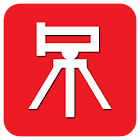Engineering Survey Calculator

• SEARCH TYPE

All Android applications categories

All Android games categories# Engineering Survey Calculator

3 10

10 Users
rating

## Screenshots

Description

Engineering Survey Calculator contains 65 Calculators and Converters, that can quickly and easily calculate and convert different Surveying and Civil Engineering parameters. Automatic & Accurate Calculations and Conversions with every Unit and Value Changes. Available in Imperial and Metric Units. Most Comprehensive Engineering Survey Calculator.

* Available in Metric and Imperial Units *

* Available in English, Français, Español, Italiano, Deutsch, Português & Nederlands *

Engineering Survey Calculator contains following 33 Calculators:
• Standard Deviation (Series of Observations)
• Probable Error (Single Observation)
• Probable Error (Combined Effects of Accidental Errors)
• Error of the Mean (Based on Combined Effects of Accidental Errors)
• Error of the Mean (Based on Specified Error of a Single Measurement)
• Specified Error of a Single Measurement
• Probable Error of the Mean

• Temperature Correction
• Measurement Correction on a Slope
• Tension Correction to Measured Length
• Sag Correction to Measured Length
• Horizontal Distance (Slope Measurements)
• Slope Correction (Slopes of 10% or less)
• Slope Correction (Slopes greater than 10%)
• Correction due to Incorrect Tape Length
• Correction due to Nonstandard Tension
• Sag Correction (Between Points of Support)

• Departure from a Level Surface
• Displacement (Horizontal Sights)
• Combined Effect of Refraction and Curvature of Earth
• Quantities of Material to be Excavated/Filled

• Relative Accuracy required between directly connected Bench Marks (First Order - Class I)
• Relative Accuracy required between directly connected Bench Marks (First Order - Class II)
• Relative Accuracy required between directly connected Bench Marks (Second Order - Class I)
• Relative Accuracy required between directly connected Bench Marks (Second Order - Class II)
• Relative Accuracy required between directly connected Bench Marks (Third Order)

• Horizontal Distance between the Instrument and the Rod (Stadia Surveying)
• Vertical Distance between the Instrument and the Rod (Stadia Surveying)
• Stadia Distance (From Instrument Spindle to Rod - Horizontal Sights)

• Photo Scale
• Photo Scale (Using Focal Length)
• Map Scale

Engineering Survey Calculator contains following 32 Converters:
• Acceleration
• Angle
• Area
• Density
• Energy/Work
• Flow Rate (Mass)
• Flow Rate (Volume)
• Fluid
• Force
• Frequency
• Hardness
• Length
• Mass
• Metric Weight
• Metrology
• Moment of Force
• Moment of Inertia
• Prefixes
• Pressure
• Specific Heat Capacity
• Specific Volume
• Temperature
• Thermal Conductivity
• Thermal Expansion
• Time
• Torque
• Velocity
• Viscosity (Dynamic)
• Viscosity (Oil & Water)
• Viscosity (Kinematic)
• Volume

Key Features:
• Complete coverage of calculators and converters in Surveying and Civil Engineering Parameters.
• Automatic Calculation & Conversion of the Output with respect to changes in the Input, Options and Units.
• Formulas are provided for each calculator.
• Extremely Accurate Calculations and Conversions.
• Professionally and Newly designed user-interface that speeds up Data Entry, Easy Viewing and Calculation Speed.

Most Comprehensive Engineering Survey Calculator

Tags: survey pack , surveying calculator , sag in engineering survey , calculating for sag in tape surveying , based survay length , engineering survey calculat , stadia calculator , survey tape temperature corrections calculator , calculate sag with survey tape

## Users review

from 3 reviews

"Awesome"

10